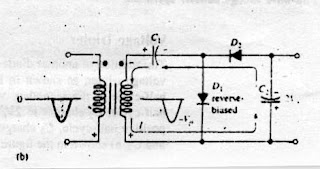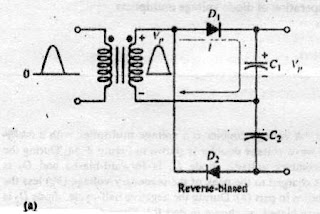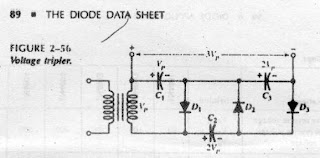Voltage Multipliers

Voltage Doubler:

Half wave Voltage Doubler:
A voltage doubler is a voltage multiplier with a multiplication factor of two.A half wave voltage doubler is shown in figure below:During the positive half-cycle of te secondary voltage,diode D1 is forward-biased and D2 is reverse-biased.Capacitor C1 is charged to the peak of the secondry voltage (Vp) less the diode drop with the polarity shown in part (a).During the negative half-cycle,diode D2 is forward-biased and D1 is reverse-biased,as shown in part (b).Since C1 can't discharge,the peak voltage on C1 adds to the secondary voltage to charge C2 to approximately 2Vp.Applying Kirchhoff's law around the loop as shown in part (b),the voltage across C2 is

Vc1-Vc2+Vp=0
Vc2=Vp+Vc1

Neglecting the diode drop of D2,Vc1=Vp,therefore,

Vc2=Vp+Vp=2Vp

Under a no load condition ,C2 remains charged to approximately 2Vp.If a load resistance is connected across the output,C2 discharges slightly through the loadon the next positive half-cycle and is again recharged to 2Vp on the following negative half-cycle.The ersulting output is a half-wave,capacitor filtered voltage.The peak inverse voltage across each diode is 2Vp.Full Wave Voltage Doubler:
A full-wave doubler is shown in figure.When the secondary voltage is positive,D1 is forward-biased and C1 charges to approximately Vp,as shown in part (a).During the negative half-cycle,D2 is forward-biased and C2 charges to approximately Vp,as shown in part (b).The output voltage,2Vp is taken across the two capacitors in series.Voltage Tripler:
The ddition of another diode-capacitor section to the half-wave voltage doubler creats a voltage tripler,as shown in figure.The operationis as follows:On the positive half-cycle of the secondary voltage,C1 charges to Vp through D1.During the negative half-cycle,C2 charges to 2Vp through D2,as described for the doubler.During the next positive half-cycle,C3 charges to 2Vp through D3.The tripler output is taken across C1 and C3,as shown in figure.